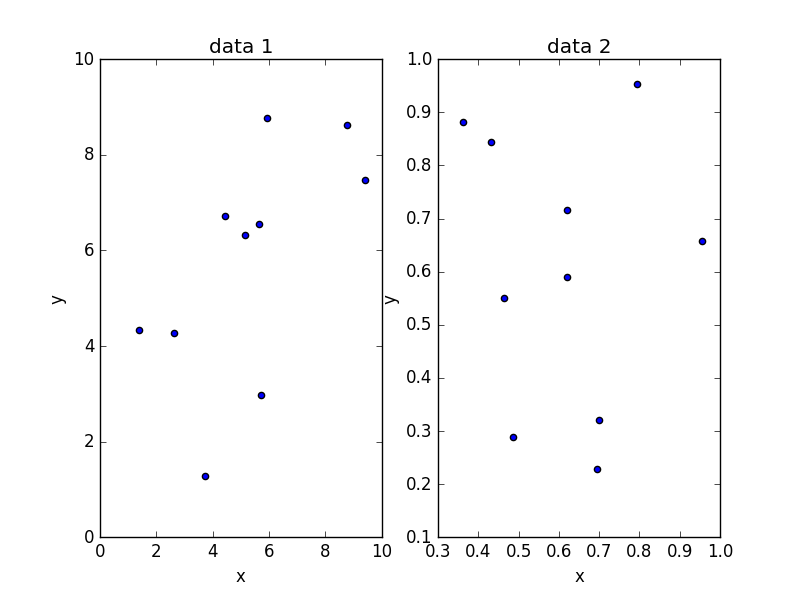# Creating multiple plots on a single figure

Through this brief introductory course, we have been plotting single plots. Multiple plots within the same figure are possible - have a look here for a detailed work through as how to get started on this - there is also some more information on how the mechanics of matplotlib actually work.

To give an overview and try and iron out any confusion, let’s run a quick example.

As when making the 3D plots, first import `matplotlib.pyplot` using an alias of `plt` and create a figure object:

``````import matplotlib.pyplot as plt
fig = plt.figure()
``````

We are going to create 2 scatter plots on the same figure. To do this we want to make 2 axes subplot objects which we will call `ax1` and `ax2`. To do this type:

``````ax1 = fig.add_subplot(121)
``````

This adds a subplot to the figure object and assigns it to a variable (`ax1` or `ax2`). The numbers - for example 121 - are a way of locating your subplot in the overall space of the figure object. The code 121 can be though of as 1 row, 2 columns, 1st position. 122 would therefore be 1 row, 2 columns, 2nd position. By defining separate axis objects, we can modify the diofferent plots specifically.

We are going to plot two basic scatter plots - create some data using numpy (import it using an alias of np):

``````import numpy as np
data_1=np.array(np.random.random((10,2)))*10
data_2=np.array(np.random.random((10,2)))
``````

We now need to define out scatter plots specifically to the axis objects of `ax1` and `ax2`, passing in the data from `data_1` and `data_2` - you can do this using:

``````ax1.scatter(data_1[:,0],data_1[:,1])
ax2.scatter(data_2[:,0],data_2[:,1])
``````

Note that we are calling the data using numpy’s indexing (look at the numpy indexing course notes here).

To modify the axis objects by adding labels, you can use the methods inherent of the axis objects e.g.:

``````ax1.set_title('data 1')
ax1.set_xlabel('x')
ax1.set_ylabel('y')

ax2.set_title('data 2')
ax2.set_xlabel('x')
ax2.set_ylabel('y')
``````

To view this, you can now just call:

``````plt.show()
``````

and you should end up with this:Have a play in the interactive plot window that opens up where you can move your data around - this also provides some options for savimng your figure. You can also save the figure (but this must be done before calling `plt.plot()`) using the plt.savefig() function.